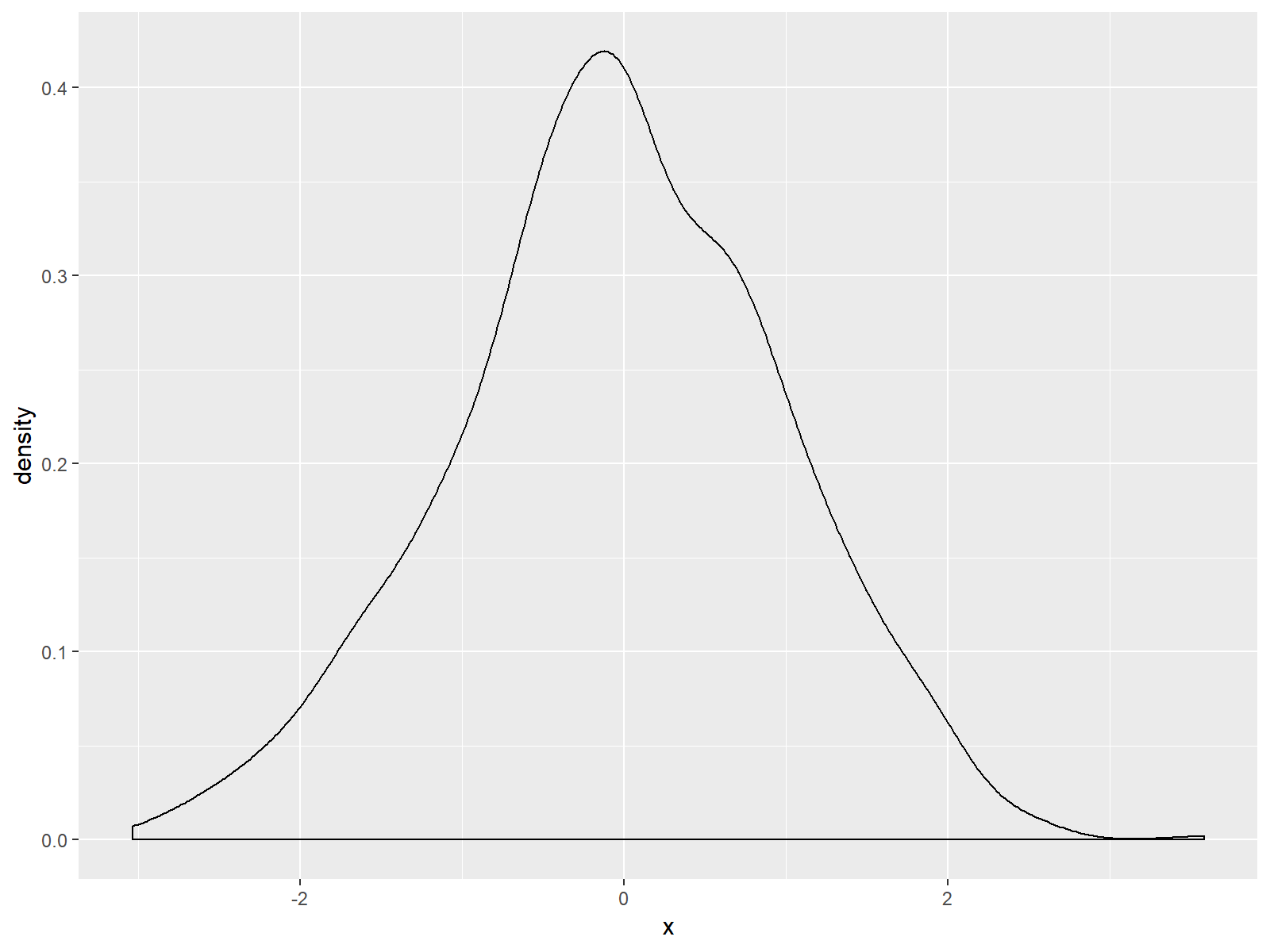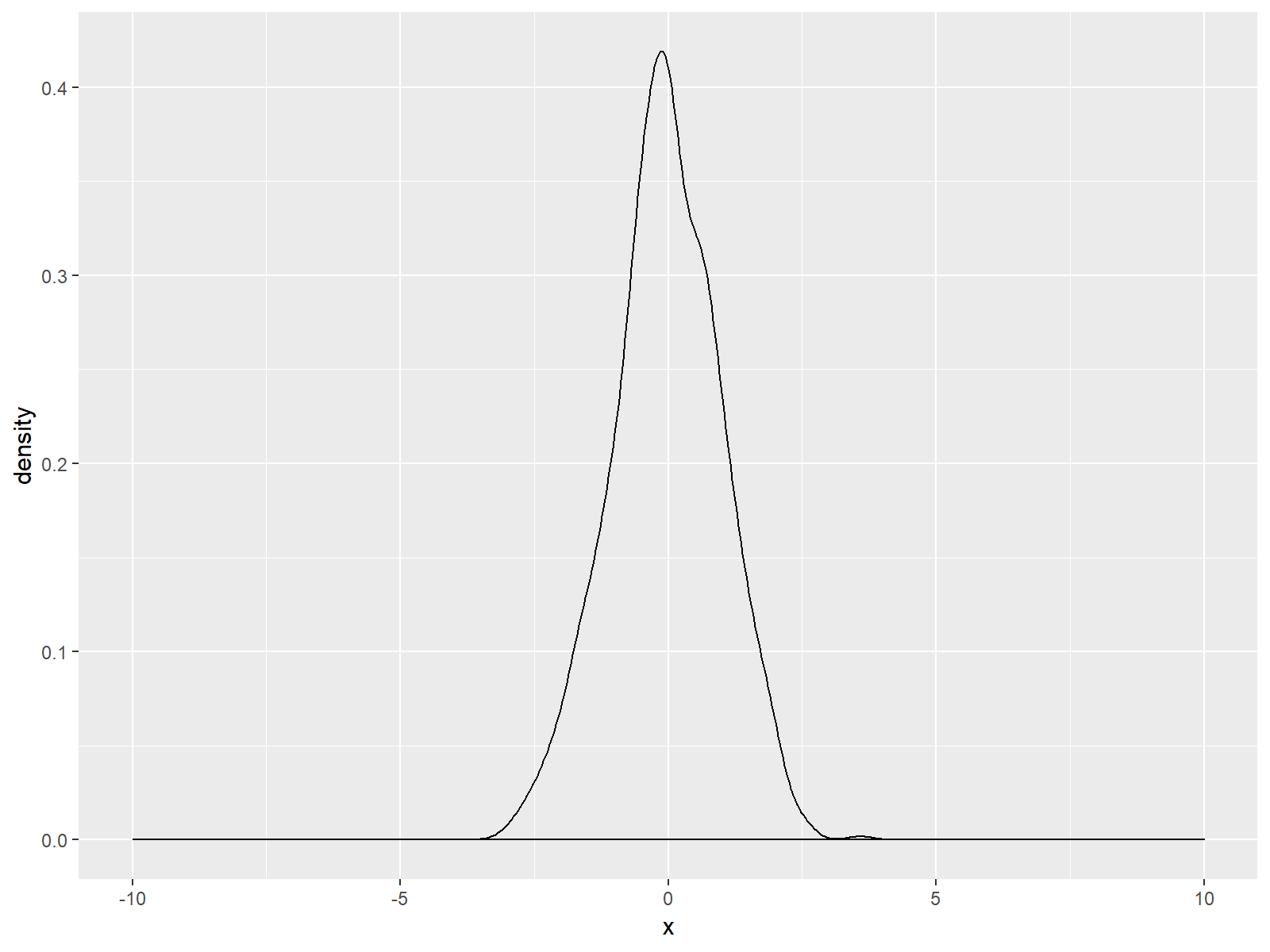# Set Axis Limits in ggplot2 R Plot (3 Examples)

This tutorial explains how to set the axis limits of a ggplot in the R programming language.

The article consists of the following contents:

Let’s get started!

## Creation of Example Data & Basic Plot

The following examples are based on this example data:

```set.seed(10101)                             # Set seed
data <- data.frame(x = rnorm(1000))         # Create example data```

To create plots with the ggplot2 package, we need to install and load the package to RStudio:

```install.packages("ggplot2")                 # Install ggplot2 package

Without any specifications of our axes, a basic density plot created with the ggplot2 package would look as follows:

```ggplot(data, aes(x = x)) +                  # Create basic density ggplot
geom_density()```Figure 1: Basic Density Plot of ggplot2 R Package.

As you can see based on Figure 1, ggplot2 automatically adjusts the axes so that all data points are shown. Furthermore the ggplot2 package leaves some space around the plotted data.

In the following examples, I’ll show you how to modify the axes of such ggplots. Note that this example is based on a density plot. However, the following R codes could be applied to any other types of plots and graphics (e.g. histogram, barchart, scatterplot, and so on…).

## Example 1: Modification of Axis Limits with scale_x_continuous

We have basically two alternatives, if we want to change our ggplot2 axis ranges. The first alternative is based on the scale_x_continuous function:

```ggplot(data, aes(x = x)) +                  # Density plot with scale_x_continuous
geom_density() +
scale_x_continuous(limits = c(-10, 10))```Figure 2: ggplot2 Density Plot with Broader x-Axis due to scale_x_continuous Function.

As you can see based on the previous R syntax, we specified the axis limits within the scale_x_continuous command to be within the range of -10 and 10. Of cause you could use any range you want.

Note: Equivalently to scale_x_continuous there also exists the scale_y_continuous function, which can be applied in the same manner in order to move the scale of the y-axis of a ggplot.

Let’s move on to the second alternative and its differences compared to Example 1…

## Example 2: Modification of Axis Limits with coord_cartesian

Another way to widen the axis ranges of our plot relies on the coord_cartesian function:

```ggplot(data, aes(x = x)) +                  # Density plot with coord_cartesian
geom_density() +
coord_cartesian(xlim = c(-10, 10))```Figure 3: ggplot2 Density Plot with Broader x-Axis due to coord_cartesian Function.

If you compare Figure 2 and Figure 3, you can see the difference between the two alternatives. The scale_x_continuous draws a black line at the bottom of our graph; but the coord_cartesian function does not.

Note: Within coord_cartesian you could also specify the ylim argument in order to adjust the y-axis.

## Example 3: Cutting off Values with Zoom In

In the previous examples, I explained how to extent the x-axis range of a ggplot. However, we can also make the shown range smaller than the default:

```ggplot(data, aes(x = x)) +                  # Zoom in
geom_density() +
scale_x_continuous(limits = c(-1, 1))
# Warning message:
# Removed 328 rows containing non-finite values (stat_density).```Figure 4: Zoomed In Density Plot is Cutting of Some Values.

As you can see based on Figure 4, we zoomed in and cut off several values of our data. This is also the reason why the RStudio console returned the following warning message:

Warning message:
Removed 328 rows containing non-finite values (stat_density)

328 observations of our example are not shown in the zoomed in version of our plot.

## Video & Further Resources

In case you need further explanations for the examples shown in this tutorial, you could have a look at the following video of the Statistics Globe YouTube channel. In the video, I’m explaining the examples of this article in more detail:

In addition, you may want to have a look at the other R tutorials of my website. You can find some interesting tutorials for handling of the ggplot2 package below. These tutorials are definitely a good basement for the data visualization with ggplot2:

In this article you learned how to set the axis limits of a ggplot in the R programming language. If you have comments or questions, don’t hesitate to let me know in the comments section below.

Subscribe to the Statistics Globe Newsletter

•Noah
May 11, 2021 5:02 am

Hi Joachim, I’m trying to scale the z-axis in a 3D plot I made using plotrgl(). I tried 2 approaches:

1) Physically removing data points in the 2-dimensional data matrix which are above/below a certain value, and then letting plotrgl() scale automatically.

2) Scaling using arguments in the plotrgl() function.

Unfortunately, I haven’t succeeded at either.

For approach 1) I followed your tutorial: https://statisticsglobe.com/remove-multiple-values-from-vector-in-r . However, the output is a vector rather than the original matrix, so information is lost. I would like to get the original matrix but with zeros for values and/or ranges which I specify.

Do you know how to implement approach 1) or approach 2) ? Both would be extremely useful for different purposes.

•May 11, 2021 7:12 am

Hey Noah,

I have not much experience with 3D plots. However, if you want to retain the matrix structure (i.e. Approach 1)), you may use a code like this:

```my_matrix <- matrix(1:12, ncol = 3) # Example matrix
my_matrix
#      [,1] [,2] [,3]
# [1,]    1    5    9
# [2,]    2    6   10
# [3,]    3    7   11
# [4,]    4    8   12

my_matrix[my_matrix %in% c(1, 5, 8)] <- 0 # Replace certain values by 0
my_matrix
#      [,1] [,2] [,3]
# [1,]    0    0    9
# [2,]    2    6   10
# [3,]    3    7   11
# [4,]    4    0   12```

Could you try if this works for your case?

Regards

Joachim

•Noah Van Horne
May 12, 2021 10:26 am

It is not exactly what I needed, because I am working with a large dataset with many thousands of elements. So I cannot replace values one at a time, and I don’t know the exact values of the elements I want to replace. I only know that I would like to remove values which are greater than some cutoff. Also, I would like to avoid filling the matrix with “0” values, because these will appear in the 3D plot.

A friend found a solution that is almost the same as yours, and gives what I need:

my_matrix 4] my_matrix
# [,1] [,2] [,3]
# [1,] 1 NaN NaN
# [2,] 2 NaN NaN
# [3,] 3 2 NaN
# [4,] 4 NaN NaN

The plotting function I am using in R is smart enough to automatically ignore the NaN values, so they are not displayed in the 3D plot.

•May 12, 2021 11:43 am

Hey Noah,

The code I have posted before is just an example on how to replace values in a matrix. You can use basically any logical condition to replace values in a matrix. To give you another example that shows how to replace values larger than 3 by NaN:

```my_matrix[my_matrix > 3] <- NaN
my_matrix
#      [,1] [,2] [,3]
# [1,]    1  NaN  NaN
# [2,]    2  NaN  NaN
# [3,]    3  NaN  NaN
# [4,]  NaN  NaN  NaN```

You can find more info on replacing values based on logical conditions in the following tutorial: https://statisticsglobe.com/replace-values-in-data-frame-conditionally-in-r

Regards

Joachim

•Noah Van Horne
May 12, 2021 10:30 am

Oops. I noticed the above code is not showing up correctly. If you email me I can send you the R script.

•May 12, 2021 11:43 am

•Noah Van Horne
May 13, 2021 1:17 am

Yes! Your line of code is the same one I tried to post. You can see the result in the example matrix above. Though in my example matrix I randomly put the value 2 in the 2nd column, 3rd row, and set the cutoff to replace values > 4 with NaN.

•May 13, 2021 6:23 am

Ah, so now it works? 🙂

•Vincenzo
February 22, 2022 7:26 am

Thanks

•March 9, 2022 11:14 am

You are very welcome Vincenzo!

•Jeff Norriss
August 25, 2022 6:43 am

Excellent explanation thanks Joachim.
However, in your examples a small length of x axis remains below and above the limits you set.
How can we trim these?

•August 25, 2022 7:42 am